## 11.3.2 Nondeterministic Finite Automata

An interesting connection lies between the ideas of this chapter and the theory of finite automata, which is part of the theory of computation (see [462,891]). In Section 2.1, it was mentioned that determining whether there exists some string that is accepted by a DFA is equivalent to a discrete feasible planning problem. If unpredictability is introduced into the model, then a nondeterministic finite automaton (NFA) is obtained, as depicted in Figure 11.8. This represents one of the simplest examples of nondeterminism in theoretical computer science. Such nondeterministic models serve as a powerful tool for defining models of computation and their associated complexity classes. It turns out that these models give rise to interesting examples of information spaces.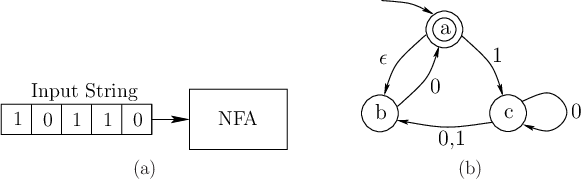An NFA is typically described using a directed graph as shown in Figure 11.8b, and is considered as a special kind of finite state machine. Each vertex of the graph represents a state, and edges represent possible transitions. An input string of finite length is read by the machine. Typically, the input string is a binary sequence of 0's and's. The initial state is designated by an inward arrow that has no source vertex, as shown pointing into state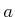in Figure 11.8b. The machine starts in this state and reads the first symbol of the input string. Based on its value, it makes appropriate transitions. For a DFA, the next state must be specified for each of the two inputs 0 andfrom each state. From a state in an NFA, there may be any number of outgoing edges (including zero) that represent the response to a single symbol. For example, there are two outgoing edges if 0 is read from state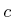(the arrow fromto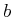actually corresponds to two directed edges, one for 0 and the other for). There are also edges designated with a special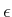symbol. If a state has an outgoing, the state may immediately transition along the edge without reading another symbol. This may be iterated any number of times, for any outgoingedges that may be encountered, without reading the next input symbol. The nondeterminism arises from the fact that there are multiple choices for possible next states due to multiple edges for the same input andtransitions. There is no sensor that indicates which state is actually chosen.

The interpretation often given in the theory of computation is that when there are multiple choices, the machine clones itself and one copy runs each choice. It is like having multiple universes in which each different possible action of nature is occurring simultaneously. If there are no outgoing edges for a certain combination of state and input, then the clone dies. Any states that are depicted with a double boundary, such as statein Figure 11.8, are called accept states. When the input string ends, the NFA is said to accept the input string if there exists at least one alternate universe in which the final machine state is an accept state.

The formulation usually given for NFAs seems very close to Formulation 2.1 for discrete feasible planning. Here is a typical NFA formulation , which formalizes the ideas depicted in Figure 11.8:

Formulation 11..2 (Nondeterministic Finite Automaton)
1. A finite state space.
2. A finite alphabet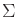which represents the possible input symbols. Let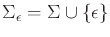.
3. A transition function,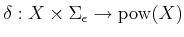. For each state and symbol, a set of outgoing edges is specified by indicating the states that are reached.
4. A start state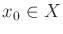.
5. A set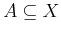of accept states.

Example 11..18 (Three-State NFA)   The example in Figure 11.8 can be expressed using Formulation 11.2. The components are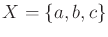,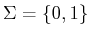,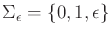,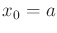, and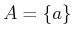. The state transition equation requires the specification of a state for every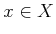and symbol in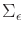: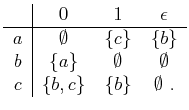(11.47)Now consider reformulating the NFA and its acceptance of strings as a kind of planning problem. An input string can be considered as a plan that uses no form of feedback; it is a fixed sequence of actions. The feasible planning problem is to determine whether any string exists that is accepted by the NFA. Since there is no feedback, there is no sensing model. The initial state is known, but subsequent states cannot be measured. The history I-state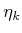at stagereduces to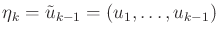, the action history. The nondeterminism can be accounted for by defining nature actions that interfere with the state transitions. This results in the following formulation, which is described in terms of Formulation 11.2.

Formulation 11..3 (An NFA Planning Problem)
1. A finite state space.
2. An action space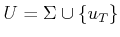.
3. A state transition function,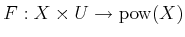. For each state and symbol, a set of outgoing edges is specified by indicating the states that are reached.
4. An initial state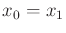.
5. A set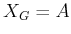of goal states.

The history I-space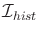is defined using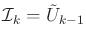(11.48)

for each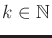and taking the union as defined in (11.19). Assume that the initial state of the NFA is always fixed; therefore, it does not appear in the definition of.

For expressing the planning task, it is best to use the nondeterministic I-space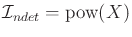from Section 11.2.2. Thus, each nondeterministic I-state,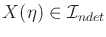, is the subset ofthat corresponds to the possible current states of the machine. The initial condition could be any subset ofbecausetransitions can occur from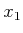. Subsequent nondeterministic I-states follow directly from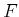. The task is to compute a plan of the form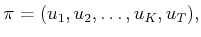(11.49)

which results in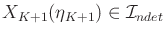with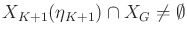. This means that at least one possible state of the NFA must lie in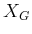after the termination action is applied. This condition is much weaker than a typical planning requirement. Using worst-case analysis, a typical requirement would instead be that every possible NFA state lies in.

The problem given in Formulation 11.3 is not precisely a specialization of Formulation 11.1 because of the state transition function. For convenience,was directly defined, instead of explicitly requiring that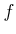be defined in terms of nature actions,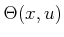, which in this context depend on bothandfor an NFA. There is one other small issue regarding this formulation. In the planning problems considered in this book, it is always assumed that there is a current state. For an NFA, it was already mentioned that if there are no outgoing edges for a certain input, then the clone of the machine dies. This means that potential current states cease to exist. It is even possible that every clone dies, which leaves no current state for the machine. This can be easily enabled by directly defining; however, planning problems must always have a current state. To resolve this issue, we could augmentin Formulation 11.3 to include an extra dead state, which signifies the death of a clone when there are no outgoing edges. A dead state can never lie in, and once a transition to a dead state occurs, the state remains dead for all time. In this section, the state space will not be augmented in this way; however, it is important to note that the NFA formulation can easily be made consistent with Formulation 11.3.

The planning model can now be compared to the standard use of NFAs in the theory of computation. A language of an NFA is defined to be the set of all input strings that it accepts. The planning problem formulated here determines whether there exists a string (which is a plan that ends with termination actions) that is accepted by the NFA. Equivalently, a planning algorithm determines whether the language of an NFA is empty. Constructing the set of all successful plans is equivalent to determining the language of the NFA.

Example 11..19 (Planning for the Three-State NFA)   The example in Figure 11.8 can be expressed using Formulation 11.2. The components are,,,, and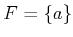. The function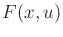is defined as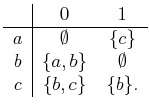(11.50)

The nondeterministic I-space is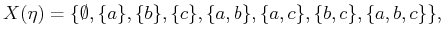(11.51)

in which the initial condition is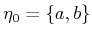because antransition occurs immediately from. An example plan that solves the problem is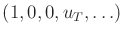. This corresponds to sending an input string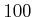'' through the NFA depicted in Figure 11.8. The sequence of nondeterministic I-states obtained during the execution of the plan is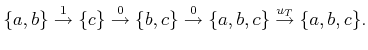(11.52)A basic theorem from the theory of finite automata states that for the set of strings accepted by an NFA, there exists a DFA (deterministic) that accepts the same set . This is proved by constructing a DFA directly from the nondeterministic I-space. Each nondeterministic I-state can be considered as a state of a DFA. Thus, the DFA has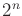states, if the original NFA hasstates. The state transitions of the DFA are derived directly from the transitions between nondeterministic I-states. When an input (or action) is given, then a transition occurs from one subset ofto another. A transition is made between the two corresponding states in the DFA. This construction is an interesting example of how the I-space is a new state space that arises when the states of the original state space are unknown. Even though the I-space is usually larger than the original state space, its states are always known. Therefore, the behavior appears the same as in the case of perfect state information. This idea is very general and may be applied to many problems beyond DFAs and NFAs; see Section 12.1.2

Steven M LaValle 2012-04-20